# ML Theory: Conditional Probability 101

Venn diagrams are useful tools for visualizing different probability spaces. Consider two events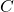and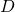within some sample space S. Supposeis the event of owning a cat andis the event of owning a dog. Our Venn diagram might look something like the following for a sample of 450 pet owners.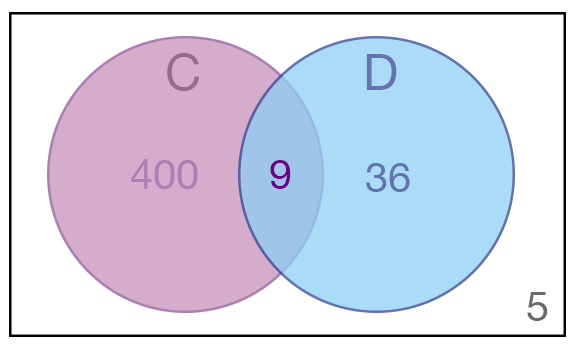Venn diagram of a sample of 450 pet owners. Here, is the event of an individual owing a cat and is the event of an individual owing a dog.

In other words, out of a sample of 450 pet owners,

• 400 owned a cat exclusively,
• 36 owned a dog exclusively,
• 9 owned both a cat and a dog, and
• 5 owned neither a cat nor a dog.

Obviously, the probability of owning a cat,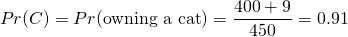is much higher than the probability of owning a dog,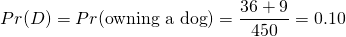as cats are the superior animal. We can also estimate the probability of being one of those people with neither a cat nor a dog as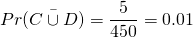Now, suppose we select a cat owner at random. What is the probability that they are also a dog owner? Well, since we know the individual is a cat owner, we only need to focus on the left hand bubble of our Venn diagram.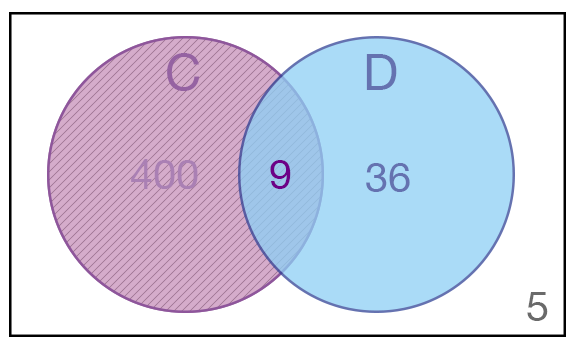The number of individuals of interest to us is therefore 409, which we will denote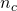. Of those 409, only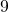are within the intersection (denoted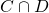). We will refer to these 9 as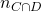. The relevant probability can therefore be estimated as,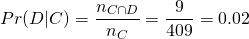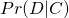represents the probability an individual owns a dog given they have a cat. This is what we refer to as a conditional probability. When calculating a conditional probability, we use additional information to reduce the size of our probability space. In this example, this meant that we could focus solely on the cat owner portion of the pictured Venn diagram.

In general, for two events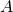and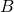, the law of conditional probability states that the probability of observinggiven eventcan be represented as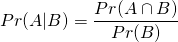This is exactly the formula we were using for the cats and dogs example,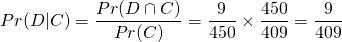We often use conditional probability in our everyday lives without thinking about it. For example, if you tested positive for an extremely rare disease, you should be thinking that the test is likely inaccurate rather than you have the disease… or maybe not because you might be freaking out right now. However, your doctor should be! As a result, your doctor would send you for further testing.